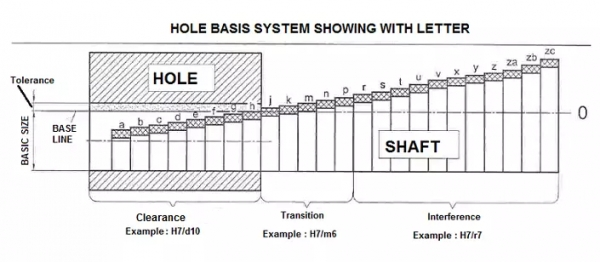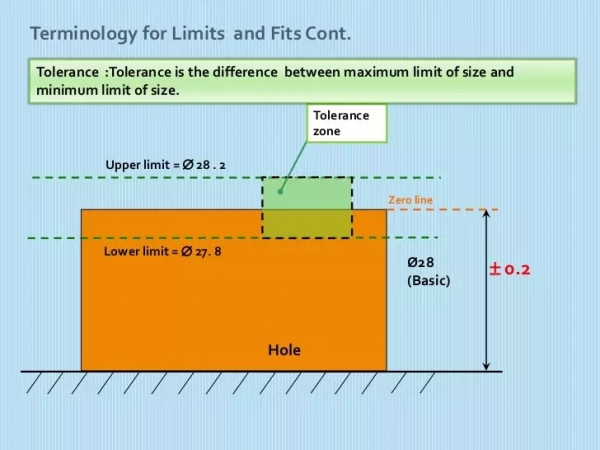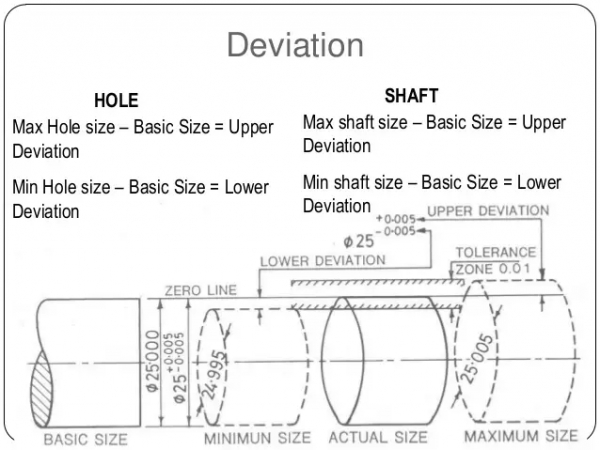# What is the basic terminology in the system of limits and fits?

Science & TechnologyPhysicsGeneral KnowledgeGeneral Topics

In the basic terminology in the system of limits and fits are a number of general definitions and terms which are used in the system of limits and fits.

The terminologies are as follows:

• Shaft: Shaft can be defined as it is a member which can fit into another part with proper assembly of the part. A shaft can either rotate or be stationary. The shaft will be applied to a member which has to fit even if there is a restriction between two spaces.• Hole: Hole can be defined as a member who can house or fit the shaft. A hole can also rotate or be stationary. A hole can be applied to space between two restrictions in which a member has to fit. If an assembly is made of two parts whether both of them are similar or not similar, one part is known as a male part (outer elements of the part), and the other part is known as female part (inner element of the part) surface. The female part is known as the hole and the male part is known as the shaft.

• Basic size: Basic size is the size which is calculated by the design engineer. This design engineer makes sure it withstands the stresses which are formed in a component. It is the same for both hole and shaft. Basic size can also be called as nominal size.

• Actual size: Actual size is the size, when manufacturing the dimensions are obtained only after completing the product. It may be per standard required dimensions or per basic required dimensions. The size of the manufactured component is called actual size.• Maximum limit: The Maximum limit is also known as a high limit, it is the maximum size permitted for the part.

• Minimum Limit: The Minimum limit is also known as a low limit, it is the minimum size permitted for the part.

• Zero lines: It is a line along which represents the basic size and zero (or initial point) for measurement of upper or lower deviations. Basic Size: It is the size with reference to which upper or lower limits of size are defined.

• Zero lines: Zero lines can be defined as the graphical representation of Zero in limits and fits. The line of zero deviation represents the basic size.• Deviation: Deviation can be defined as the algebraic difference between the actual manufactured size and the basic size. Deviations can happen when the product is being manufactured. Utmost care is required for the product.

• Upper Deviation: Upper deviation can be defined as the algebraic difference between the maximum limit of size and the corresponding basic size.

• Lower Deviation: Lower deviation can be defined as the algebraic difference between the minimum limit of size and the corresponding basic size.